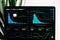# How to add Exponential Moving Averages to Your Trading Arsenal

## Step-by-step examples using Python

Moving average indicators are used in a variety of trading strategies to spot long-term trends in the price data. One potential drawback of simple moving average strategies is that they weight all of the prices equally, whereas you might want more recent prices to take on a greater importance. The exponential moving average (EMA) is one way to accomplish this.Photo by Luke Chesser on Unsplash

# TL;DR

We walk through the EMA calculation with code examples and compare it to the SMA.

# Calculating the Exponential Moving Average

The EMA gives more weight to the most recent prices via a weighting multiplier. This multiplier is applied to the last price so that it accounts for a larger chunk of the moving average than the other data points.

The EMA is calculated by taking the most recent price (we’ll call it 𝑃𝑡, or “price at time 𝑡”) and subtracting the EMA from the previous time period (𝐸𝑀𝐴𝑡−1). This difference is weighted by the number of time periods you set your EMA to (𝑁) and added back to the 𝐸𝑀𝐴𝑡−1.

Mathematically, we can write it like this:

You may have noticed that the above equation has a slight problem, how does it get started? It’s referencing the last period’s EMA, so if you go to the first calculation, what is it referencing? This is usually alleviated by substituting the simple moving average (SMA) to initialize the calculation so that you can build the EMA for all time periods after the first.

Let’s show how this works with a simple example in Python by importing our packages.

`import numpy as npimport pandas as pdimport yfinance as yfimport matplotlib.pyplot as plt`

From here, we will build two functions to work together and calculate our indicator. The first function will be a simple implementation of the formula we outlined above:

`def _calcEMA(P, last_ema, N):    return (P - last_ema) * (2 / (N + 1)) + last_ema`

The second function will calculate the EMA for all of our data, first by initializing it with the SMA, then iterating over our data to update each subsequent entry with the value in our SMA column or calling the `_calcEMA` function we defined above for rows greater than N.

`def calcEMA(data, N):    # Initialize series    data['SMA_' + str(N)] = data['Close'].rolling(N).mean()    ema = np.zeros(len(data))    for i, _row in enumerate(data.iterrows()):        row = _row        if i < N:            ema[i] += row['SMA_' + str(N)]        else:            ema[i] += _calcEMA(row['Close'], ema[i-1], N)    data['EMA_' + str(N)] = ema.copy()    return data`

Now, let’s get some data and see how this works. We’ll pull a shorter time period than we would use for a backtest and compare 10, 50, and 100 days of the EMA and SMA.

`ticker = 'GM'yfObj = yf.Ticker(ticker)data = yfObj.history(ticker, start='2018-01-01', end='2020-12-31')N = [10, 50, 100]_ = [calcEMA(data, n) for n in N]colors = plt.rcParams['axes.prop_cycle'].by_key()['color']fig, ax = plt.subplots(figsize=(18, 8))ax.plot(data['Close'], label='Close')for i, n in enumerate(N, 1):    ax.plot(data[f'EMA_{n}'], label=f'EMA-{n}', color=colors[i])    ax.plot(data[f'SMA_{n}'], label=f'SMA-{n}', color=colors[i],        linestyle=':')ax.legend()ax.set_title(f'EMA and Closing Price Comparison for {ticker}')plt.show()`

You can see in the plot above that the EMAs are more responsive to recent changes than the SMAs. Shorter time horizons too are even more responsive than longer time horizons which have a price “memory” that may stretch back months or more.

Moving averages of all types are lagging indicators meaning they only tell you what has already happened in the price. However, this doesn’t mean they can’t be useful for identifying trends and developing strategies that use one or more moving average indicators.

One common approach is to use moving averages to look for cross-overs — points where a faster indicator moves above or below a slower indicator to generate signals. Another possibility includes using these indicators as market level filters to adjust risk exposure.

If you have an idea, go ahead and test it out, see how EMA, SMA, and other values can be combined to develop new and profitable trading strategies.

At Raposa, you can quickly test your ideas with no code and high-quality data to run backtests. Find a strategy that works for you and deploy it to get live trading alerts.

## Raposa Technologies

Bringing Quant Finance to the People

Written by

## Raposa Technologies

Bringing Quant Finance to You

## Raposa Technologies

We are building new applications to enable no-code solutions to enable you to trade like a pro. Follow us for the latest developments, research, and strategies.

Written by

## Raposa Technologies

Bringing Quant Finance to You## Raposa Technologies

We are building new applications to enable no-code solutions to enable you to trade like a pro. Follow us for the latest developments, research, and strategies.

Medium is an open platform where 170 million readers come to find insightful and dynamic thinking. Here, expert and undiscovered voices alike dive into the heart of any topic and bring new ideas to the surface. Learn more

Follow the writers, publications, and topics that matter to you, and you’ll see them on your homepage and in your inbox. Explore

If you have a story to tell, knowledge to share, or a perspective to offer — welcome home. It’s easy and free to post your thinking on any topic. Write on Medium